### Home > CALC > Chapter Ch10 > Lesson 10.1.5 > Problem10-46

10-46.
1. Find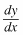for the following equations. Homework Help ✎

1.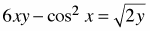2.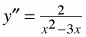3.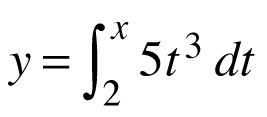4. y = ln(ln(ln x))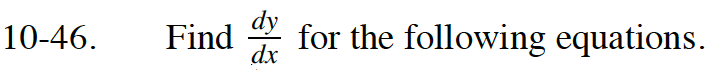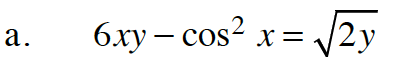Differentiate:

$6y+6xy^\prime-2\cos(x)(-\sin(x))=\frac{1}{2}(2y)^{-1/2}(2y^\prime)$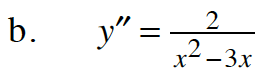Since y″ is given, integrate.

Use partial fraction decomposition:

$\frac{2}{x^2-3x}=\frac{a}{x}+\frac{b}{x-3}$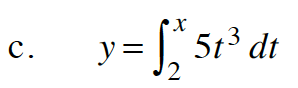This will be the derivative of an integral, so apply the Fundamental Theorem of Calculus.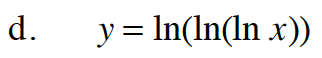Apply the Chain Rule twice.